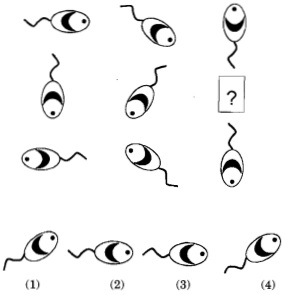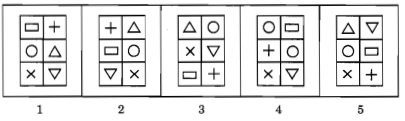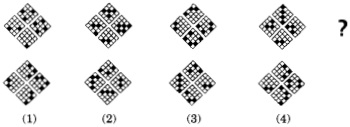# Non Verbal Reasoning Questions and Answers Part 5

17. What comes next?a. 1
b. 2
c. 3
d. 4

Explanation:

First column both figures have 5 dots on left side. 2nd column has 4 dots on left side.
3rd column first figure has 6 dots so second figure too must have 6 dots.
So answer is figure 2 i.e. option b

18. What comes in place of question mark?a. 1
b. 2
c. 3
d. 4

Explanation:

Consider one column at a time. Fishes are moving 90° clockwise.
Also, their tail inverts every time they move by 90°.
Move the fish by 90° and then invert the tail.

19. Out of the given 5 images, one image is not same as the other 4. Which is it?a. 3
b. 4
c. 2
d. 5

Explanation:

Figure 4 has 2 circles instead of 2 triangles.

20. What will be next in the sequence?a. 1
b. 2
c. 3
d. 4